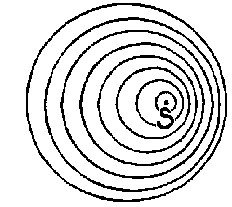• FAQ
• Contact/
/
/
1) A string fixed at both ends that vibrating in
Not my Question
Flag Content

# Question :   1) A string fixed at both ends that vibrating in : 2043704

1) A string fixed at both ends that is vibrating in its eighth harmonic will have 8 antinodes and 9 nodes.

2) A guitar string is fixed at both ends.  If you tighten it to increase its tension, the frequencies of its normal modes will increase but its wavelengths will not be affected.

3) If a tube filled with air and closed at both ends is 1.60 m long, the longest possible wavelength of the standing waves in this tube is 1.60 m.

4) If the fourth harmonic for a string fixed at both ends is 220 Hz, the fundamental frequency for this string is 880 Hz.

5) If one speaker emits a wave crest at the same time that another one emits a wave trough, these speakers are π/2 out of phase with each other.

6) Two speakers next to each other oscillate in phase, one at 404 Hz and the other at 400 Hz. If you stand in front of them, you will hear a sound of frequency 402 Hz that fluctuates between loud and soft with a frequency of 4 Hz.

7) A string 2.0 m long and fixed at both ends is vibrating in its fourth harmonic in a room at 20C. The wavelength of the sound it produces is 1.0 m.

8) A tube open at one end and closed at the other produces sound of fundamental frequency of 350 Hz. If you now open the closed end, the fundamental frequency becomes 175 Hz.

9) A thin film of refractive index 1.40 covers a flat sheet of glass of refractive index 1.50. If light of wavelength 600 nm strikes the film from air perpendicular to its surface, the minimum film thickness needed to cause destructive interference in the reflected light is 150 nm.1) In the figure above, some wavefronts are emitted by a source of sound S. This picture can help us to understand

A) why the siren on a police car changes its pitch as it races past us.

B) why a sound grows quieter as we move away from the source.

C) how sonar works.

D) the phenomenon of beats.

E) why it is that our hearing is best near 3000 Hz.

2) When a guitar is tuned, what is it that is changed?

A) The wavelength of the fundamental.

B) The frequency of the fundamental.

C) The amplitude of the fundamental.

3) Which of the following is an accurate statement?

A) A system like a vibrating string has only one possible frequency.

B) In order for a singer to break a wine glass by singing, she must adjust the amplitude of the sound she makes so that it is exactly equal to the amplitude of vibration of the wine glass.

C) The fundamental frequency of a system is the name given to the highest possible frequency at which the system will naturally vibrate.

D) The sound in an organ pipe can vibrate at an infinite number of frequencies.

## Solution 5 (1 Ratings )

Solved
Chemistry 1 Year Ago 148 Views